﻿ 视听觉跨模态表面材质检索
«上一篇文章快速检索 高级检索

 智能系统学报2019, Vol. 14Issue (3): 423-429  DOI: 10.11992/tis.2018040300

### 引用本文LIU Zhuokun, LIU Huaping, HUANG Wenmei, et al. Audiovisual cross-modal retrieval for surface material[J]. CAAI Transactions on Intelligent Systems, 2019, 14(3): 423-429. DOI: 10.11992/tis.201804030.### 文章历史

1. 河北工业大学 省部共建电工装备可靠性与智能化国家重点实验室，天津 300130;
2. 清华大学 智能技术与系统国家重点实验室，北京 100084

Audiovisual cross-modal retrieval for surface material
LIU Zhuokun 1, LIU Huaping 2, HUANG Wenmei 1, WANG Bowen 1, SUN Fuchun 2
1. State Key Laboratory of Reliability and Intelligence of Electrical Equipment, Hebei University of Technology, Tianjin 300130, China;
2. State Key Lab of Intelligent Technology and Systems, Tsinghua University, Beijing 100084, China
Abstract: Text and image features sometimes do not allow for true and accurate analysis of the material. To solve this problem, a cross-modal method for surface material retrieval in an audiovisual field is proposed. First, the sound feature is extracted using mel frequency cepstral coefficients (MFCCs), and the image feature is extracted using convolutional neural network (CNN). Then, these two features are mapped to the subspace using canonical correlation analysis and are further retrieved via Euclidean distance. Experimental validation performed using the tactile texture dataset of the Technical University of Munich showed that the proposed method has a good application effect on material retrieval.
Key words: cross-modal retrieval    feature extraction    canonical correlation analysis    subspace mapping    material analysis    convolutional neural network    Mel-frequency cepstral coefficients    Euclidean distance

1 跨模态检索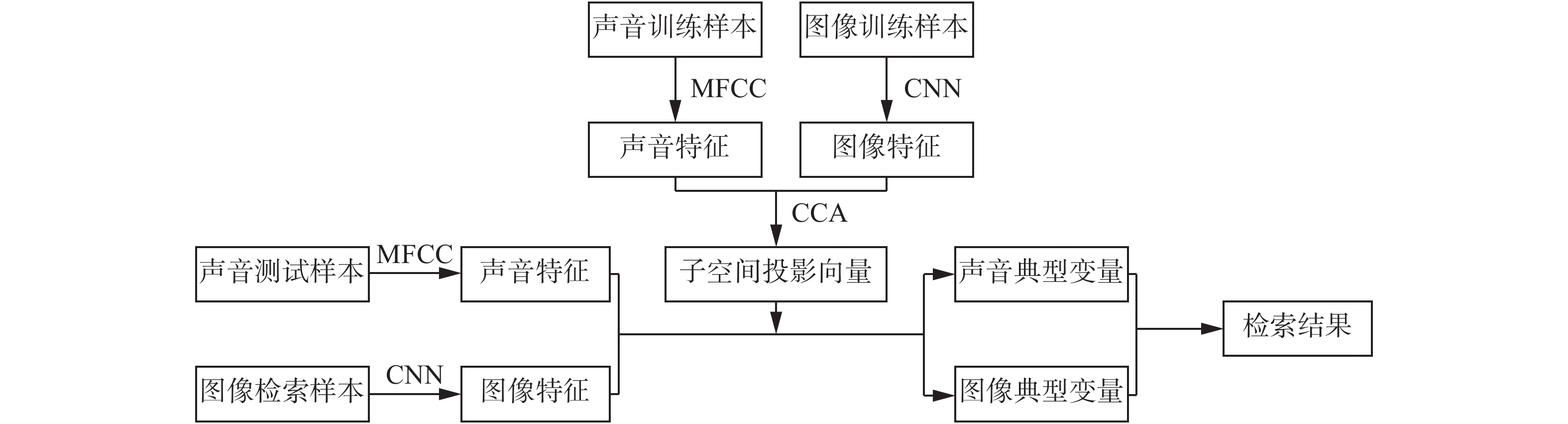Download: 图 1 检索流程 Fig. 1 The retrieval process
2 声音和图像特征提取

2.1 梅尔频率倒谱系数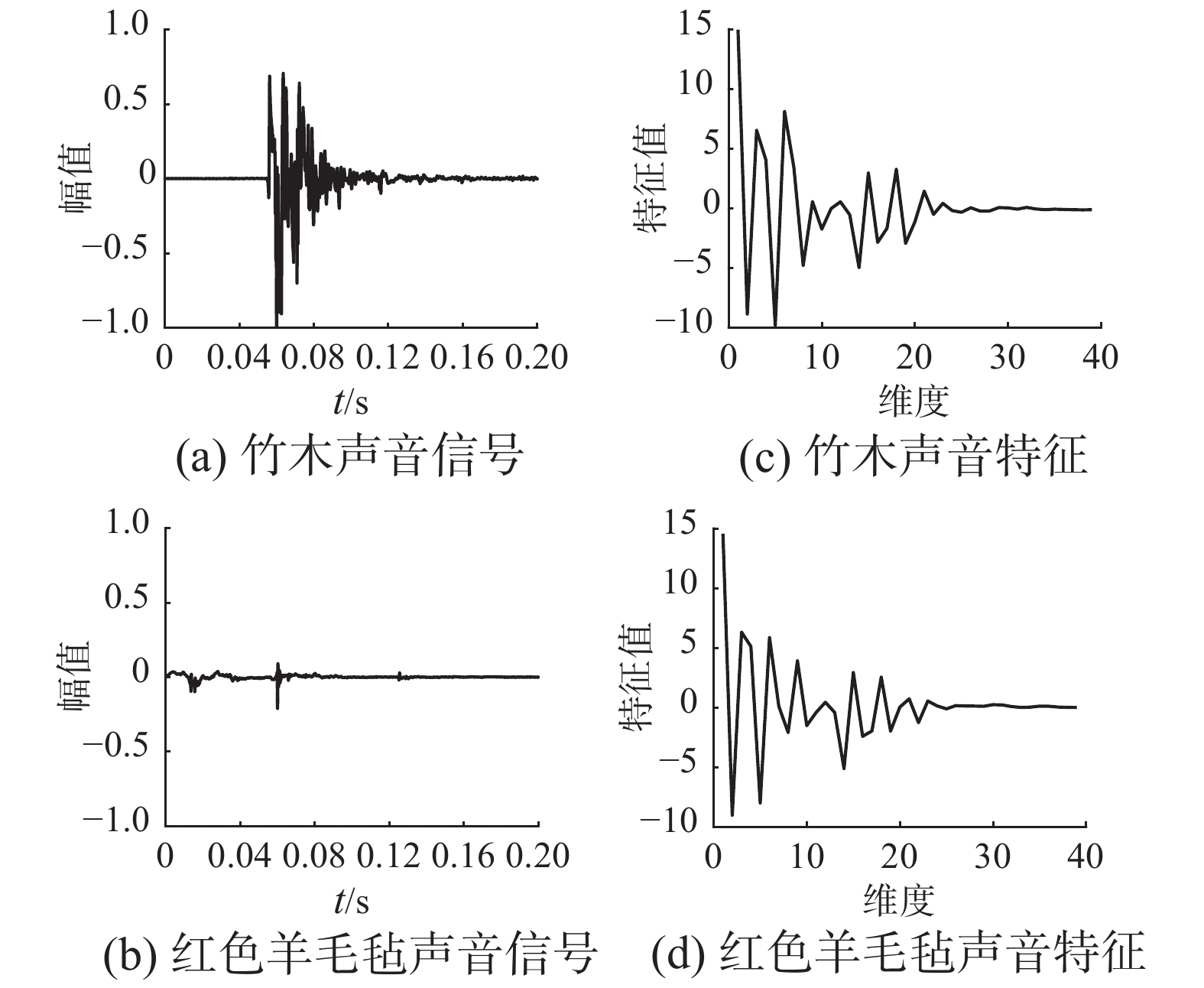Download: 图 2 竹木和红色羊毛毡声音信号和声音特征 Fig. 2 Sound signals and features of bamboo and red fleece
2.2 卷积神经网络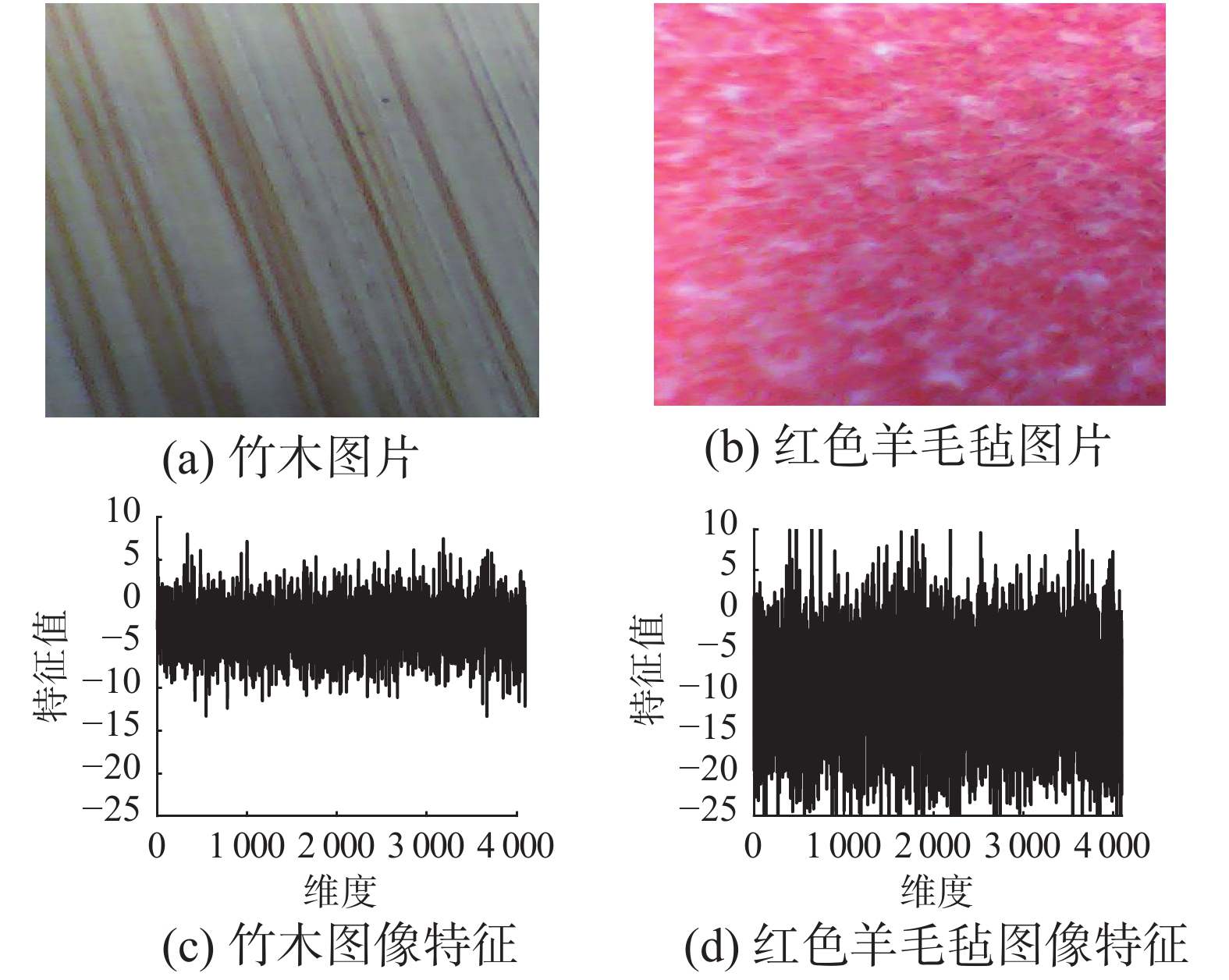Download: 图 3 竹木和红色羊毛毡图片和图像特征 Fig. 3 Image features of bamboo and red fleece
3 典型相关分析

3.1 典型相关分析基本原理

 $\rho = \frac{{{{{\omega}} _{{x}}}^{\rm{T}}{{{\varSigma }}_{{{XY}}}}{{{\omega}} _{{y}}}^{\rm{T}}}}{{\sqrt {({{{\omega}} _{{x}}}^{\rm{T}}{{{\varSigma }}_{{{XX}}}}{{{\omega}} _{{x}}})} \sqrt {({{{\omega}} _{{y}}}^{\rm{T}}{{{\varSigma }}_{{{YY}}}}{{{\omega}} _{{y}}})} }}$ (1)

 ${{{\varSigma }}_{{{XY}}}}{\rm{ = }}\frac{{\rm{1}}}{n}\sum\limits_{p{\rm{ = 1}}}^n {{{{x}}_p}{{y}}_p^{\rm{T}}}$
 ${{{\varSigma }}_{{{XX}}}}{\rm{ = }}\frac{{\rm{1}}}{n}\sum\limits_{p = 1}^n {{{{x}}_p}{{x}}_p^{\rm{T}}}$
 ${{{\varSigma }}_{{{YY}}}}{\rm{ = }}\frac{{\rm{1}}}{n}\sum\limits_{p = 1}^n {{{{y}}_p}{{y}}_p^{\rm{T}}}$

 $L = {{{\omega}} _{{x}}}^{\rm{T}}{{{\varSigma }}_{{{XY}}}}{{{\omega}} _{{y}}} - \frac{\lambda }{2}({{{\omega}} _{{x}}}^{\rm{T}}{{{\varSigma }}_{{{XX}}}}{{{\omega}} _{{x}}} - 1) - \frac{\theta }{2}({{{\omega}} _{{y}}}^{\rm{T}}{{{\varSigma }}_{{{YY}}}}{{{\omega}} _{{y}}} - 1)$ (2)

 ${{\varSigma }}_{{{XX}}}^{ - 1}{{{\varSigma }}_{{{XY}}}}{{\varSigma }}_{{{YY}}}^{ - 1}{{{\varSigma }}_{{{YX}}}} = {\lambda ^2}{{\omega}}$ (3)
3.2 改进的典型相关分析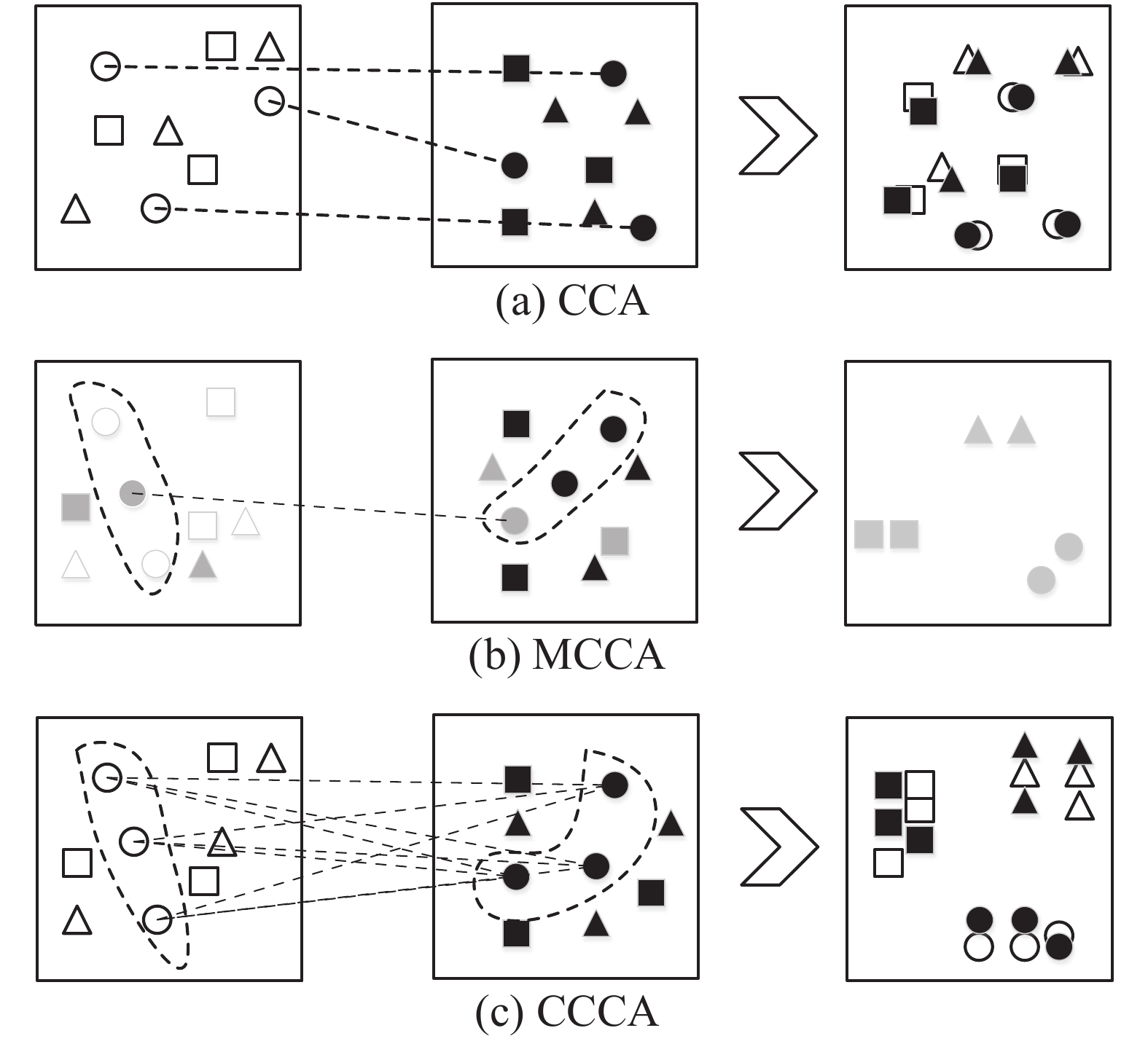Download: 图 4 3种方法的子空间对应关系 Fig. 4 The subspace correspondences of the three methods

 ${{{X}}_c} = [x_{\rm{1}}^{{c}}\;x_{\rm{2}}^{{c}}\cdots x_{\left| {{X_c}} \right|}^{{c}}]$
 ${{{Y}}_c} = [y_{\rm{1}}^{{c}}\;y_{\rm{2}}^{{c}} \cdots y_{\left| {{Y_c}} \right|}^{{c}}]$

3.2.1 均值典型相关分析

 $\rho = \frac{{{{{\omega}} _{{x}}}^{\rm{T}}{{{M}}_{{{XY}}}}{{{\omega}} _{{y}}}^{\rm{T}}}}{{\sqrt {({{{\omega}} _{{x}}}^{\rm{T}}{{{M}}_{{{XX}}}}{{{\omega}} _{{x}}})} \sqrt {({{{\omega}} _{{y}}}^{\rm{T}}{{{M}}_{{{YY}}}}{{{\omega}} _{{y}}})} }}$ (4)

 ${{{M}}_{{{XY}}}}{\rm{ = }}\frac{{\rm{1}}}{C}\sum\limits_{c = 1}^C {{{\mu}} _x^c(} {{\mu}} _y^c{)^{\rm{T}}}$
 ${{{M}}_{{{XX}}}}{\rm{ = }}\frac{{\rm{1}}}{C}\sum\limits_{c = 1}^C {{{\mu}} _x^c(} {{\mu}} _x^c{)^{\rm{T}}}$
 ${{{M}}_{{{YY}}}}{\rm{ = }}\frac{{\rm{1}}}{C}\sum\limits_{c = 1}^C {{{\mu}} _y^c(} {{\mu}} _y^c{)^{\rm{T}}}$

3.2.2 聚类典型相关分析

 $\rho = \frac{{{{{\omega}} _{{x}}}^{\rm{T}}{{{R}}_{{{XY}}}}{{{\omega}} _{{y}}}^{\rm{T}}}}{{\sqrt {({{{\omega}} _{{x}}}^{\rm{T}}{{{R}}_{{{XX}}}}{{{\omega}} _{{x}}})} \sqrt {({{{\omega}} _{{y}}}^{\rm{T}}{{{R}}_{{{YY}}}}{{{\omega}} _{{y}}})} }}$ (5)

 ${{{R}}_{{{XY}}}}{\rm{ = }}\frac{{\rm{1}}}{T}\sum\limits_{c = 1}^C {\sum\limits_{i = 1}^{\left| {{{{X}}_c}} \right|} {\sum\limits_{j = 1}^{\left| {{{{Y}}_c}} \right|} {{{x}}_i^c{{({{y}}_j^{\rm{c}})}^{\rm{T}}}} } }$
 ${{{R}}_{{{XX}}}}{\rm{ = }}\frac{{\rm{1}}}{T}\sum\limits_{c = 1}^C {\sum\limits_{i = 1}^{\left| {{{{X}}_c}} \right|} {\left| {{{{Y}}_c}} \right|{{x}}_i^c{{({{x}}_i^{{c}})}^{\rm{T}}}} }$
 ${{{R}}_{{{YY}}}}{\rm{ = }}\frac{{\rm{1}}}{T}\sum\limits_{c = 1}^C {\sum\limits_{j = 1}^{\left| {{{{Y}}_c}} \right|} {\left| {{{{X}}_c}} \right|{{y}}_j^c{{({{y}}_j^{{c}})}^{\rm{T}}}} }$

4 实验结果及分析Download: 图 5 数据集中包含的所有材料 Fig. 5 Materials included in the data set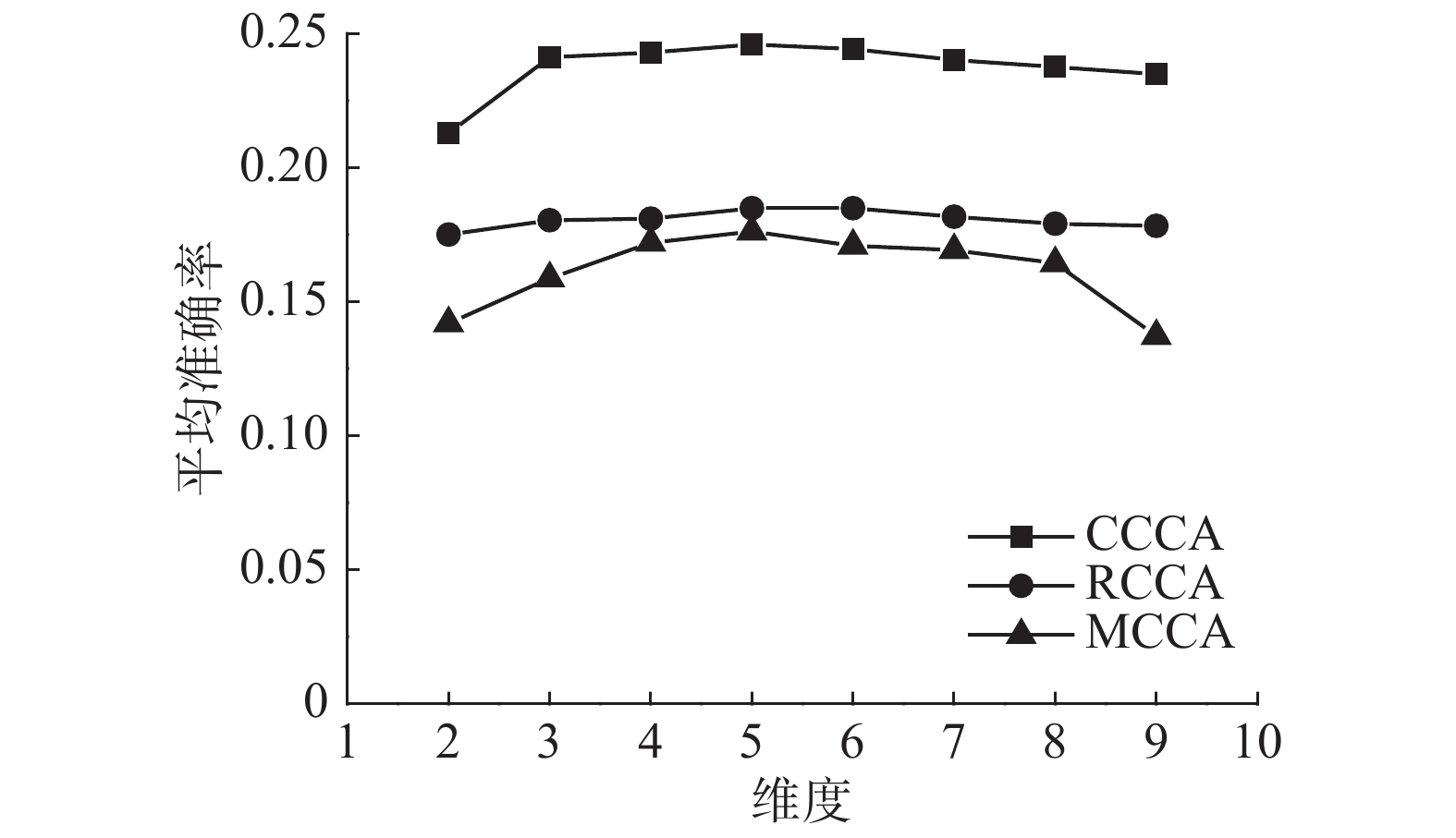Download: 图 6 不同方法的MAP值随子空间维度的变化 Fig. 6 Variation of the MAP of different methods with subspace dimensions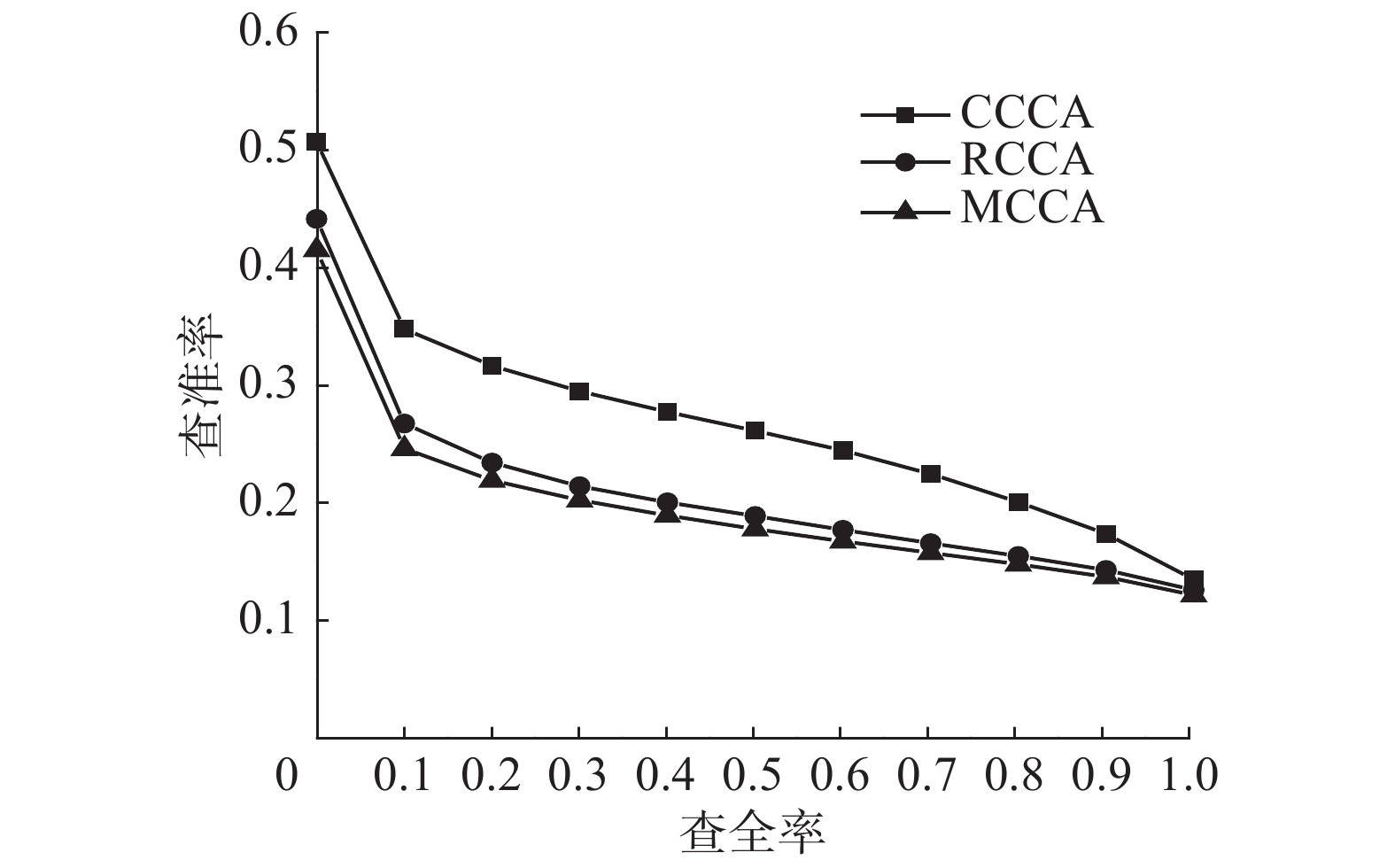Download: 图 7 PR曲线 Fig. 7 PR curveDownload: 图 8 3种材料的低维映射图 Fig. 8 Low-dimensional mapping of three materials表 1 不同材质类别的MAP Tab.1 MAP of different categories of material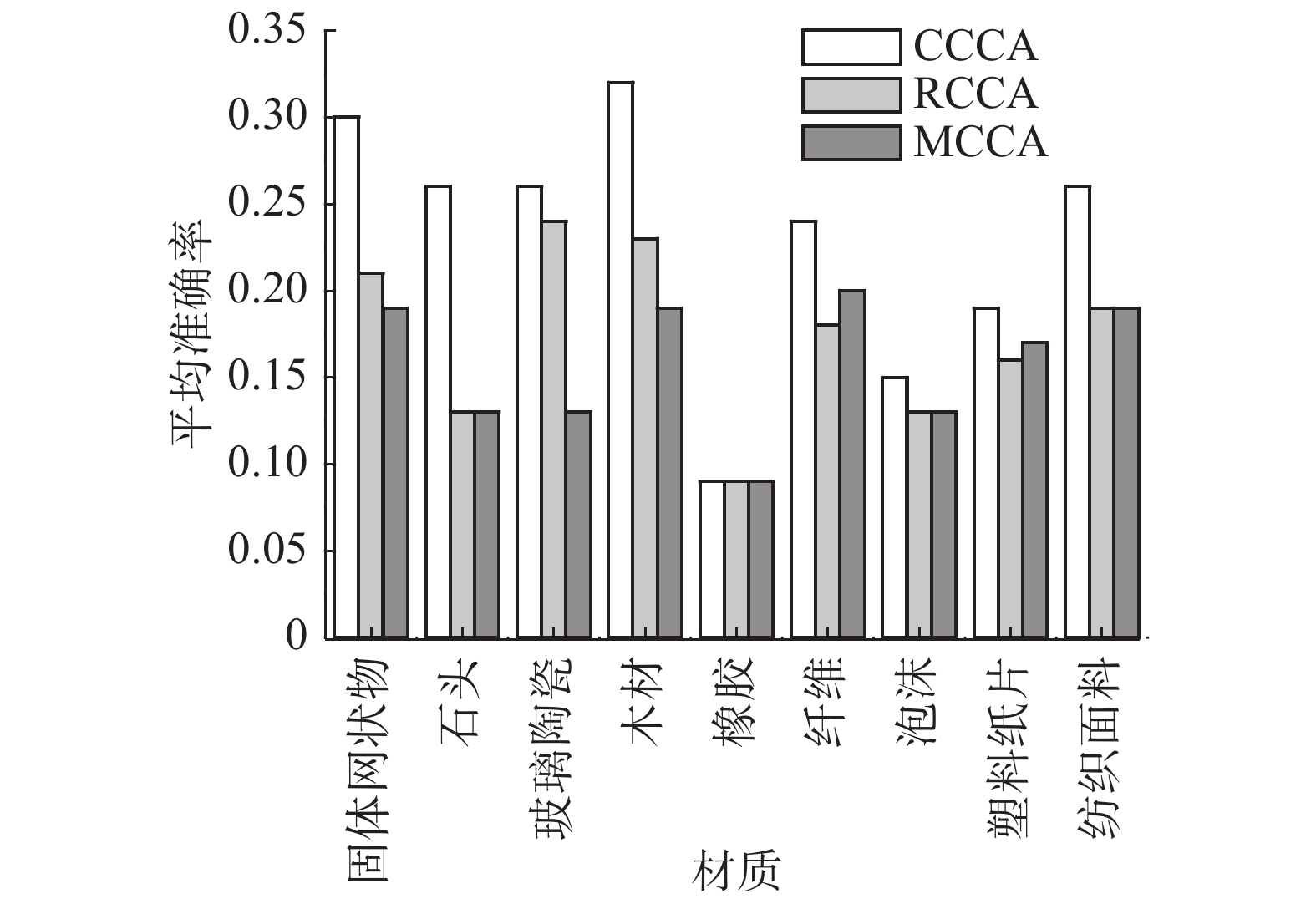Download: 图 9 不同材质类别的MAP Fig. 9 MAP of different categories of materialDownload: 图 10 使用竹木声音样本的检索结果 Fig. 10 Retrieval result of bamboo sound sample
5 结束语

  MANDAL D, BISWAS S. Query specific re-ranking for improved cross-modal retrieval[J]. Pattern Recognition Letters, 2017, 98: 110-116. DOI:10.1016/j.patrec.2017.09.008 (0)  WANG Kaiye, HE Ran, WANG Liang, et al. Joint feature selection and subspace learning for cross-modal retrieval[J]. IEEE transactions on pattern analysis and machine intelligence, 2016, 38(10): 2010-2023. DOI:10.1109/TPAMI.2015.2505311 (0)  DENG Cheng, TANG Xu, YAN Junchi, et al. Discriminative dictionary learning with common label alignment for cross-modal retrieval[J]. IEEE transactions on multimedia, 2016, 18(2): 208-218. DOI:10.1109/TMM.2015.2508146 (0)  ZHANG Liang, MA Bingpeng, LI Guorong, et al. Metric based on multi-order spaces for cross-modal retrieval[C]//Proceedings of 2017 IEEE International Conference on Multimedia and Expo. Hong Kong, China, 2017: 1374-1379. (0)  张毅, 谢延义, 罗元, 等. 一种语音特征提取中Mel倒谱系数的后处理算法[J]. 智能系统学报, 2016, 11(2): 208-215. ZHANG Yi, XIE Yanyi, LUO Yuan, et al. Postprocessing method of MFCC in speech feature extraction[J]. CAAI transactions on intelligent systems, 2016, 11(2): 208-215. (0)  WEI Yunchao, ZHAO Yao, LU Canyi, et al. Cross-modal retrieval with CNN visual features: a new baseline[J]. IEEE transactions on cybernetics, 2017, 47(2): 449-460. (0)  RANJAN V, RASIWASIA N, JAWAHAR C V. Multi-label cross-modal retrieval[C]//Proceedings of 2015 IEEE International Conference on Computer Vision. Santiago, Chile, 2015: 4094-4102. (0)  SHARMA A, KUMAR A, DAUME H, et al. Generalized multiview analysis: a discriminative latent space[C]//Proceedings of 2012 IEEE Conference on Computer Vision and Pattern Recognition. Providence, USA, 2012: 2160-2167. (0)  HARDOON D R, SZEDMAK S, SHAWE-TAYLOR J. Canonical correlation analysis: an overview with application to learning methods[J]. Neural Computation, 2004, 16(12): 2639-2664. DOI:10.1162/0899766042321814 (0)  CHEN Yongming, WANG Liang, WANG Wei, et al. Continuum regression for cross-modal multimedia retrieval[C]//Proceedings of the 19th IEEE International Conference on Image Processing. Orlando, USA, 2013: 1949-1952. (0)  MANDAL D, BISWAS S. Generalized coupled dictionary learning approach with applications to cross-modal matching[J]. IEEE transactions on image processing, 2016, 25(8): 3826-3837. DOI:10.1109/TIP.2016.2577885 (0)  STRESE M, SCHUWERK C, IEPURE A, et al. Multimodal feature-based surface material classification[J]. IEEE transactions on haptics, 2017, 10(2): 226-239. DOI:10.1109/TOH.2016.2625787 (0)  CAO Jiuwen, ZHAO Tuo, WANG Jianzhong, et al. Excavation equipment classification based on improved MFCC features and ELM[J]. Neurocomputing, 2017, 261: 231-241. DOI:10.1016/j.neucom.2016.03.113 (0)  RASIWASIA N, MAHAJAN D, MAHADEVAN V, et al. Cluster canonical correlation analysis[C]//Proceedings of the Seventeenth International Conference on Artificial Intelligence and Statistics. Reykjavik, Iceland, 2014: 823-831. (0)  STRESE M, BOECK Y, STEINBACH E. Content-based surface material retrieval[C]//Proceedings of 2017 IEEE World Haptics Conference. Munich, Germany, 2017: 352-357. (0)